# Trig Identities: Which Ones You Should and Which Ones You Shouldn’t Memorize

Any student who has taken trigonometry, precalculus, or calculus is familiar with the list of trigonometric function identities that many teachers require to be memorized - our private Anaheim online math tutors are here to help.  Sines, Cosines, Tangents, Secants, Cosecants, and Cotangents are coupled with Thetas, Alphas, and Betas in a long list of equations that students are expected to remember.  See an example list of identities below:These lists and the new math vocabulary that they use is often very daunting to students. Memorizing them all is quite the task, especially as there are many places to get mixed up (e.g., remembering if the negative sign comes first or second, remembering where the alphas and betas go, and confusing cosecant and secant).

Fortunately for students, if you read this post or have a good math teacher, you won’t have to memorize them all. Many of them can be derived quickly using others. While it would be convenient to know every trigonometric identity off the top of your head, there are better things that math students should be spending their time on.

Unfortunately for students, you will have to memorize some of them. Trigonometry introduces new math vocabulary, and you simply have to know what the words mean if you are going to use them successfully. There are also proofs that cannot be quickly or easily derived and will have to be memorized if your class requires you to use them without an equation sheet.

Let’s go through the list, starting with the tangent identities.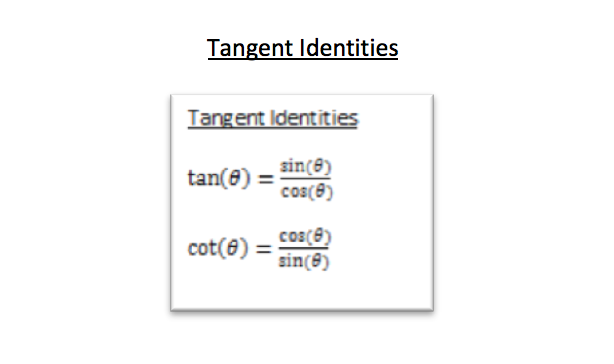The tangent identities you should memorize. They are used very often and are fundamental to trigonometry.  However, you don’t have to make yourself memorize them since you probably already know enough to derive them both.  You likely have learned SOH-CAH-TOA, the mnemonic used to identify the appropriate sides of a right triangle when working with trigonometric functions.  You may also have even learned CHO-SHA-CAO, the version for secant, cosecant, and tangent.

SOH represents that the Sine of an angle is equal to the side Opposite that angle divided by the Hypotenuse of the triangle.CAH represents that the Cosine of an angle is equal to the side Adjacent to that angle divided by the Hypotenuse of the triangle.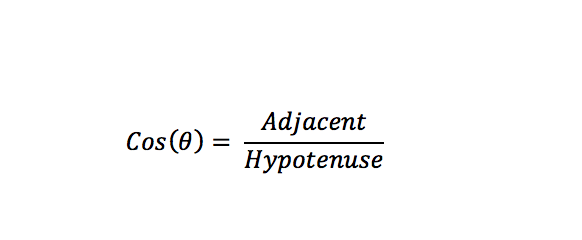Now, if we look at our first tangent identity, we see that tangent is supposed to be equal to sine divided by cosine.  But we actually already knew that from SOH-CAH-TOA.  Look what happens if we put SOH divided by CAH: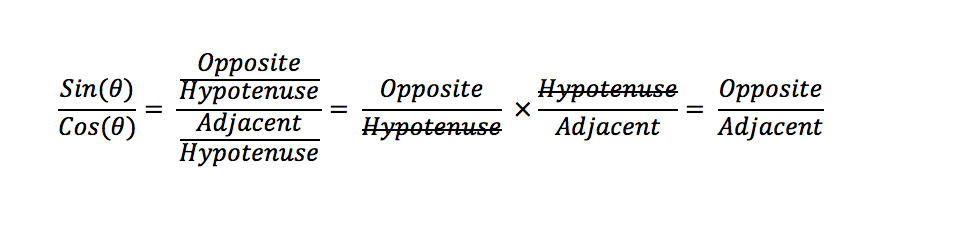Dividing by a fraction is the same as multiplying by the reciprocal of the denominator.  When we do this, we notice that the “Hypotenuse” cancels out, leaving with only Opposite over Adjacent.  Conveniently, what’s the last part of our SOH-CAH-TOA?

TOA represents that the Tangent of an angle is equal to the side Opposite that angle divided by the side Adjacent to the angle.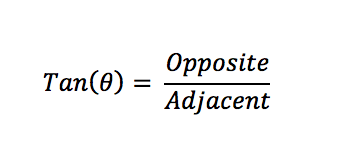We have the same result from the mnemonic. This is a very simple derivation using information you have already learned, though you will probably just get used to learning that tangent is sine over cosine anyway.

The same can be done to find the cotangent identity using CHO-SHA-CAO, or you can figure out that cotangent is the reciprocal of the tangent identity by using our next identities, the reciprocal identities: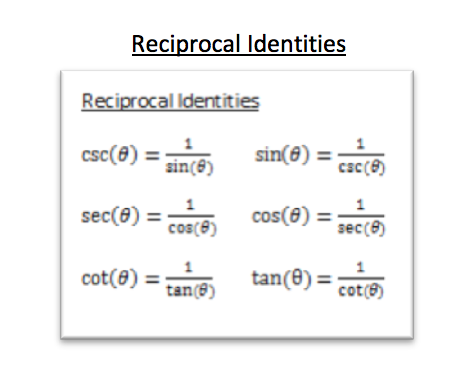Notice here that cotangent is equal to the reciprocal of tangent (and vice-versa).  This means that the two tangent identities above are really only one identity.  If you know that tangent is sine over cosine, and you know that cotangent is the reciprocal of a tangent, then you can conclude that cotangent is cosine over sine.

The reciprocal identities do have to be memorized. These are fewer identities than they are definitions. Cosecant, secant, and cotangent are known as the reciprocal trigonometric functions because they are the reciprocals of the basic three.  You need to know that cosecant is the reciprocal of sine (i.e., one divided by sine). This is just what the cosecant function is.

Unfortunately, you will have to remember that Co-secant goes with Sine, but Secant goes with Co-sine.

We’ll tackle the remaining six classes of trigonometric identities coming up!